30 Sep

The inspiration for this blog post is a plain breakfast bagel, as pictured below in this photo taken in Winthrop Dining Hall on a Sunday morning. The bagel closely resembles a shape very important in the topics of geometry and topology in mathematics–that is, the torus.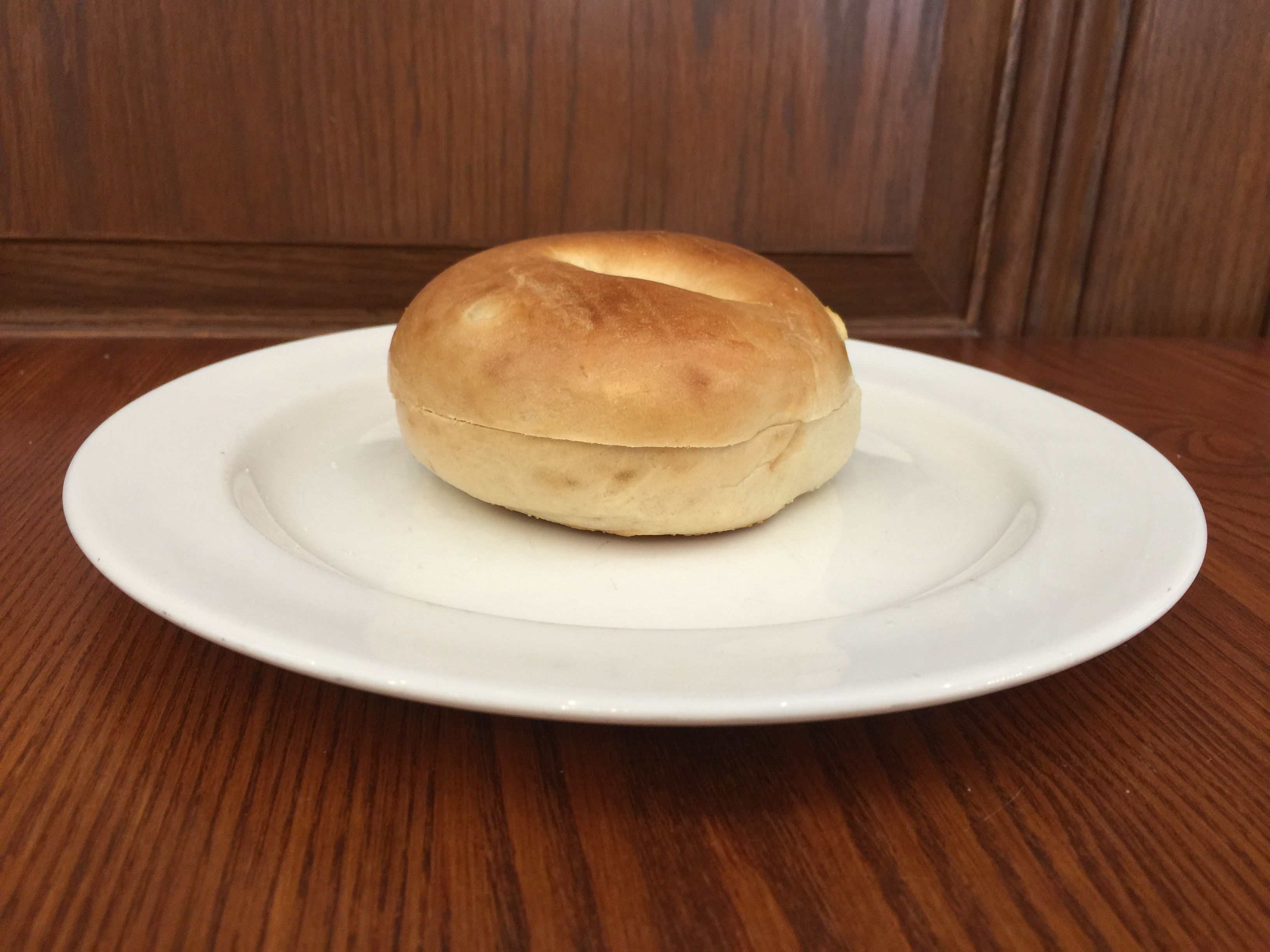The Bagel and the Torus
The torus is a surface which you can picture as follows: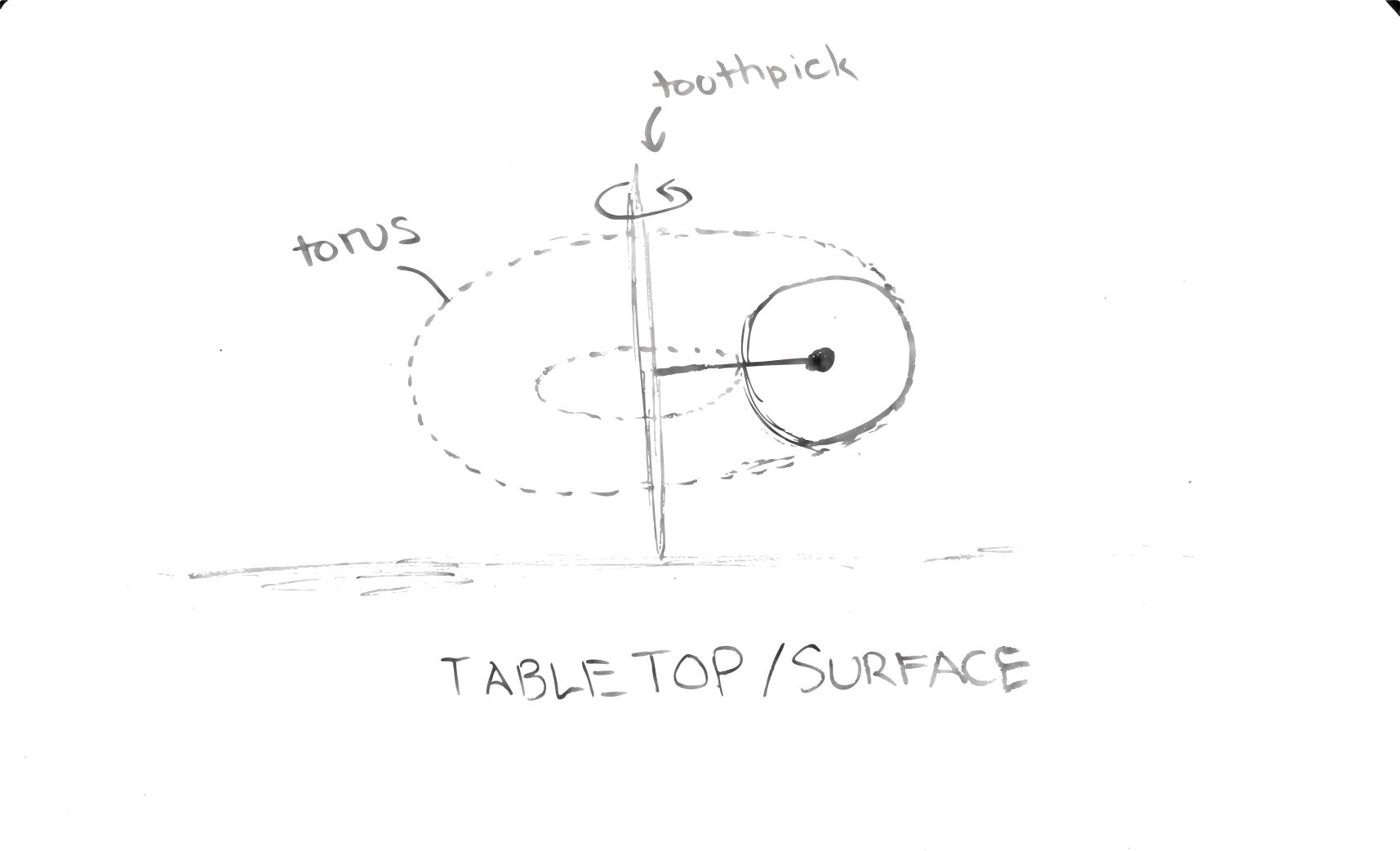Tori can live in any n-dimensional space for n>2, but because humans view bagels in 3-D, this discussion will center around the torus constructed in 3-dimensional space.

One way to visualize the torus is as follows:

• Consider a toothpick held vertically against a tabletop. This will be our axis of rotation.
• For a circle drawn on the same plane as the line passing through the toothpick, the surface created by spinning the toothpick 360 degrees forms the entire torus.

However, bagels are not hollow. Therefore, the rest of this discussion will focus on the solid torus, whose surface is an ordinary torus like the one visualized in the steps above, but also includes all of the points trapped within the torus.

Even acknowledging that real bagels are imperfect and therefore only closely approximates the solid torus, we see that this seemingly mundane and rather plain breakfast item becomes a foundational example in the field of topology!

Cutting The Bagel

If you enjoy your morning bagel with cream cheese, then some interesting mathematics might come into play: How should I slice my bagel so that I can have more space to spread cream cheese?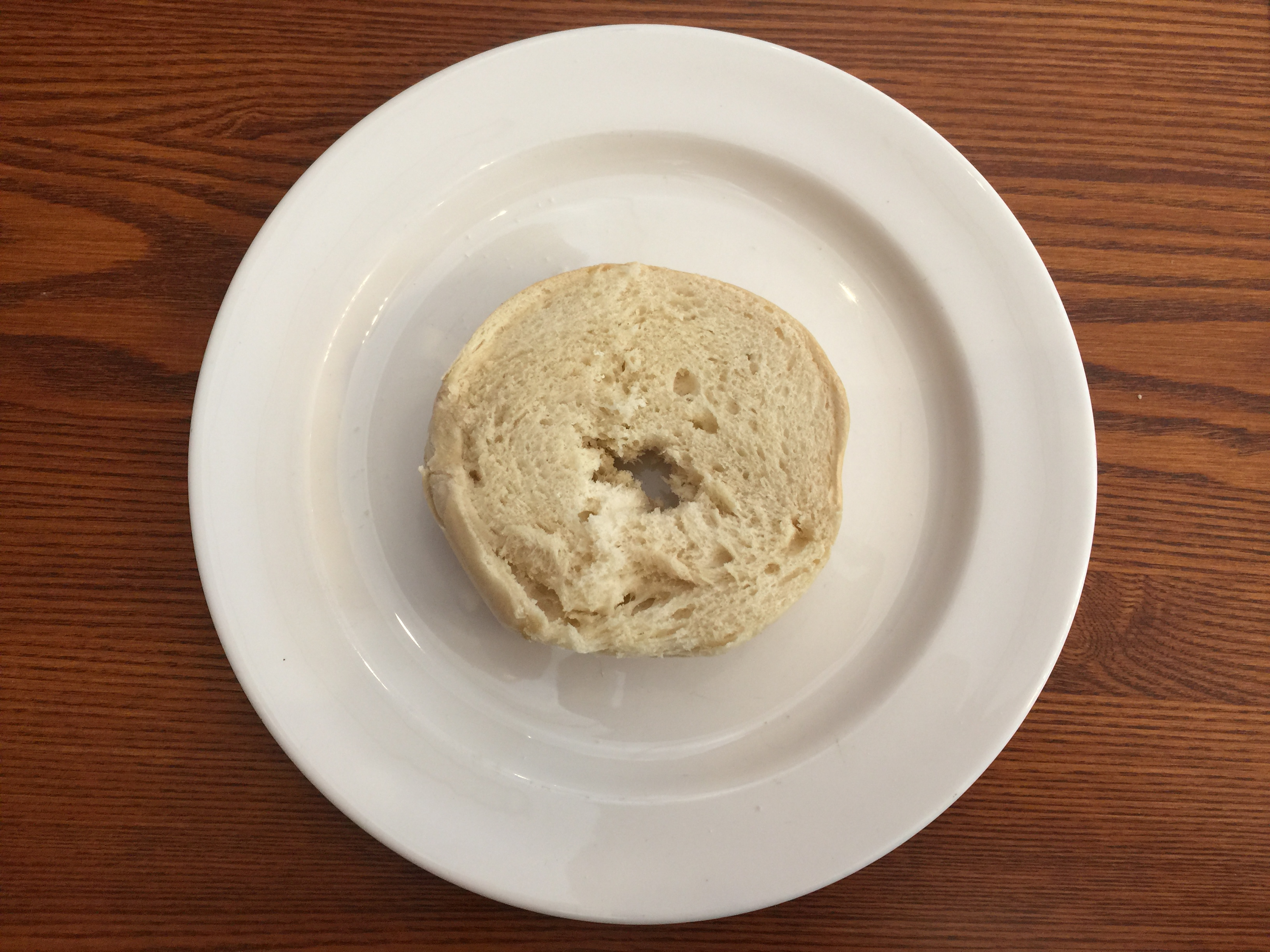Typically, the bagel is sliced horizontally to create two halves on which to spread cream cheese. Let us say we will limit the slicing to one connected motion of a knife.

What if we slice using a cut shaped like a sine wave all around the bagel? Surely that would give more surface area of exposed white bread for cream cheese.Expanding this to an extreme case, what if we keep increasing the frequency of this sine wave so that we have a near-infinite number of ridges on which to spread my cream cheese? Continuing this logic, in theory there is no limit to the amount of surface area we can generate with the cut. Therefore this question becomes difficult to answer. Perhaps the best way to maximize the surface area of exposed bagel is to just cut as many ridges as physically possible with my knife along the side of my bagel, where the limit of how thin we can slice is only physical reality.

An Interesting Cut

In 2012, Professor George W. Hart published an article about an activity he introduced to his students at Stony Brook University in which they were challenged with slicing a bagel into congruent, interlocking halves in one cut.

If you want to give this puzzle a shot, then put a hold on reading this blog post and find yourself a bagel and a knife.

.

.

.

The solution to this puzzling task is to slice the bagel along a two-twist Mobius Strip. (https://www.georgehart.com/bagel/bagel.html)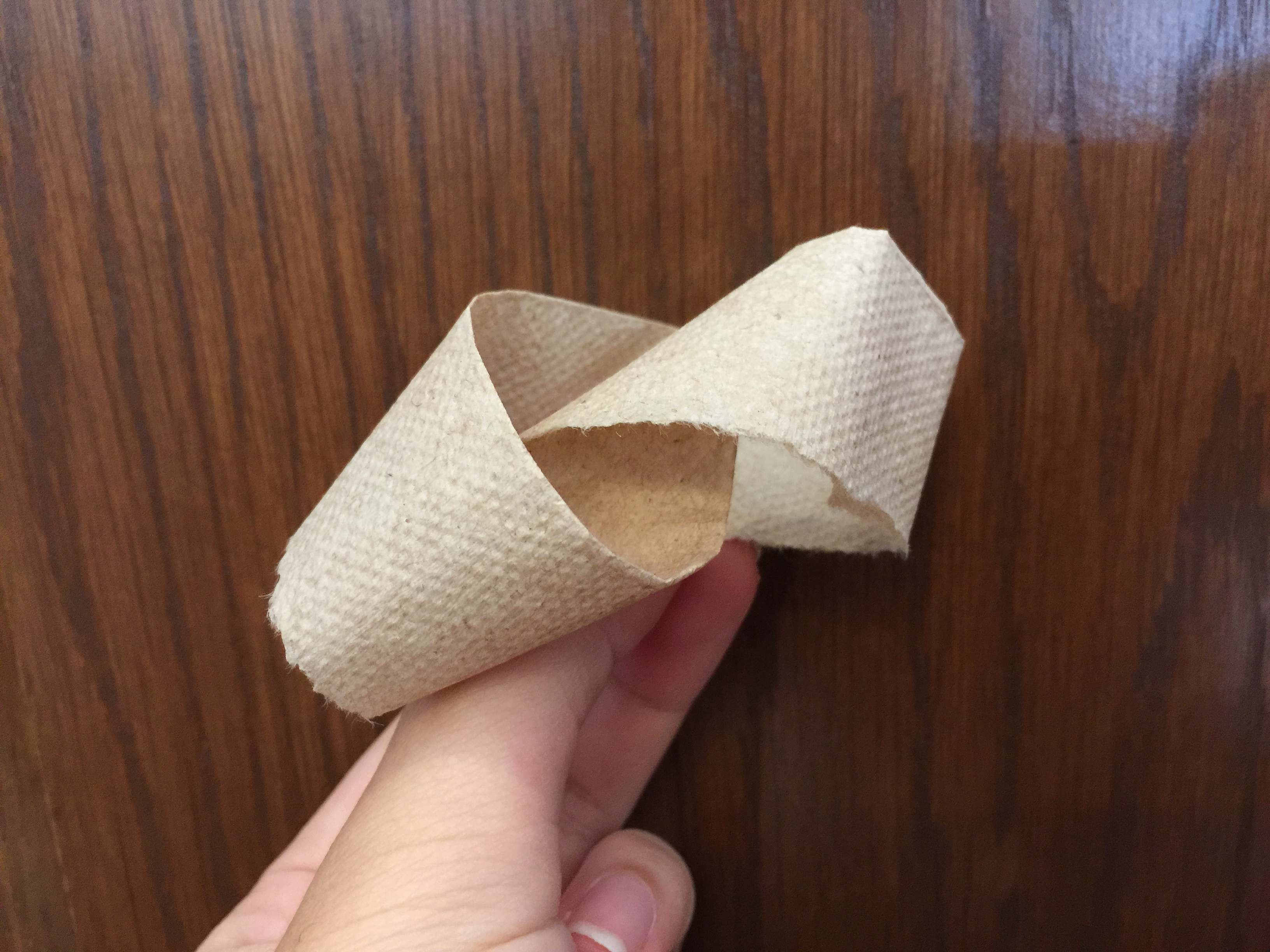*One great way to visualize a two-twist Mobius Strip is to take a strip of paper, turn one end around twice while holding the other still, and gluing the two ends together.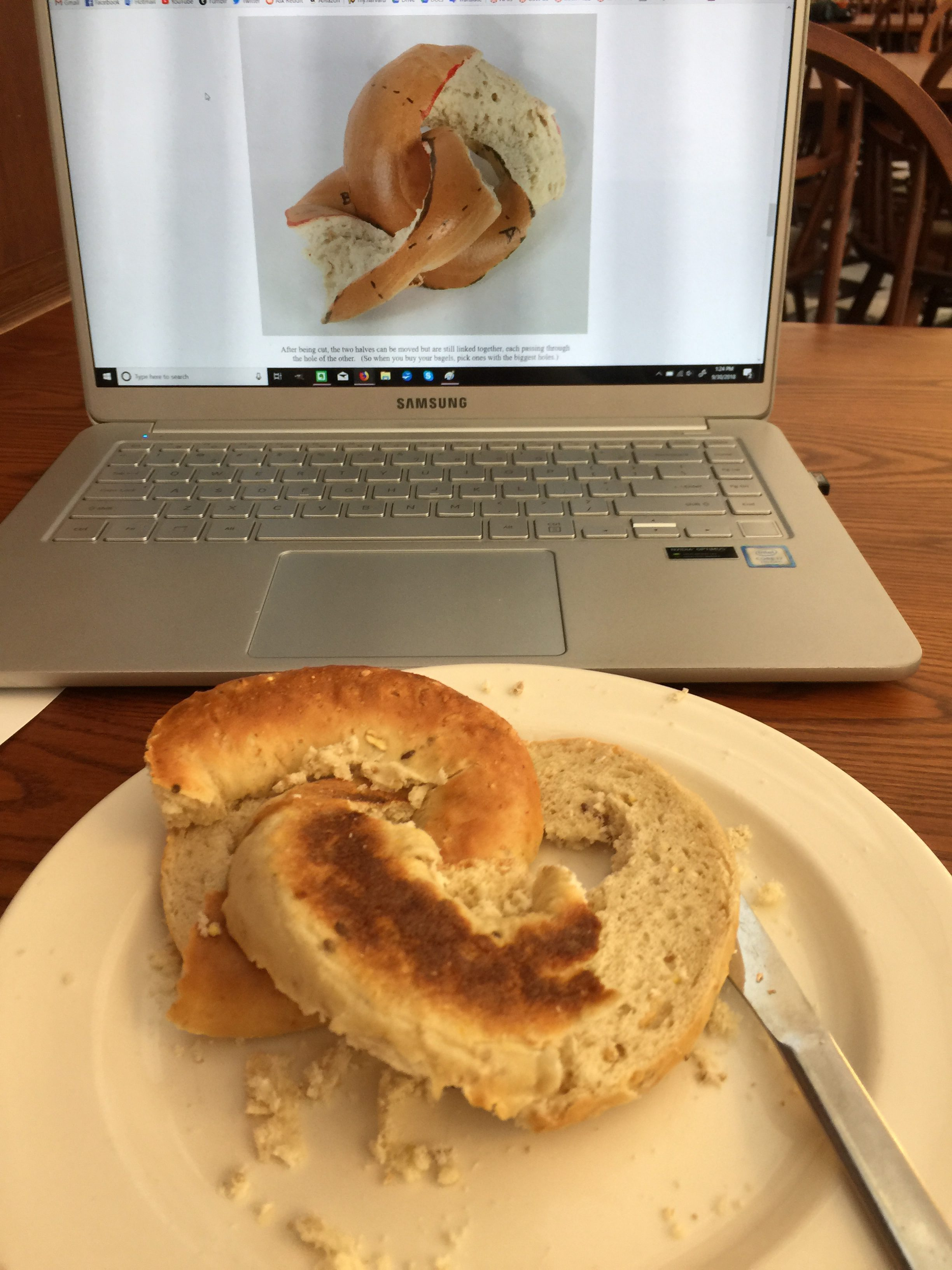I bring this up because Professor Hart’s challenge to his students is a fun and engaging activity that draws the attention of people who may never have even heard of tori and mobius strips. The idea of a single cut that splits one ring into two interlocking ones is exciting, and for that reason I will use this specific cut as an example in the exploration of surface area. Does this cut increase the amount of exposed bagel on which I can spread cream cheese? While this is surely not the most time-efficient method of cutting a bagel, we can use mathematics to test whether or not such a cut would actually give us more space on which we can slather our tasty cream cheese.

Calculating the Surface Area of Exposed Bagel in the Mobius Cut

In order to calculate the surface area of exposed bagel for our cream cheese, we will have to introduce some calculus: First, we will parametrize the torus using the following parametrization (in other words, re-representing the torus using a different set of variables):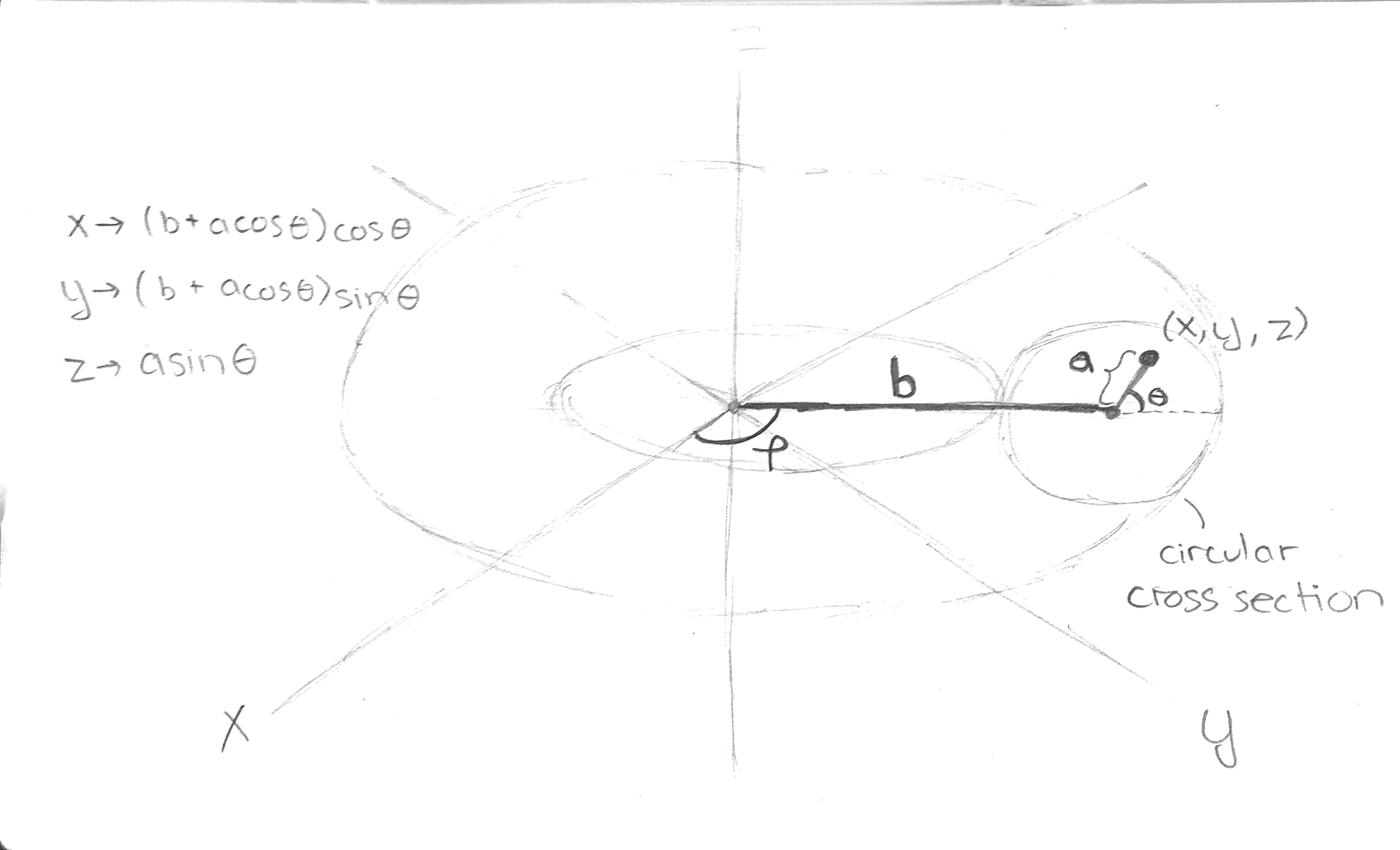The parametrization is (x,y,z) = P(a, θ,ϕ)=((acosθ+b)cosϕ, (acosθ+b)sinϕ, asinθ), where a is the distance of the point from the center of the circle that we rotate and b is the distance of the center of that circle to the center of the torus (which our toothpick passes through). The value θ tells us the angle between the segment connecting the point (x,y,z) and the center of the circle, and ϕ tells us how far we’ve rotated the toothpick (in other words, which disk cross section).

Second, we will consider the slice: a two-twist mobius strip. This slice includes all the points along a diameter of each circle. Furthermore, θ varies equally with ϕ, so we set up a parametrization for the mobius slice as follows:

(x,y,z) = M(r, θ) = ((rcosθ+b)cosθ, (rcosθ+b)sinθ, rsinθ) where a varies from -a to a and θ varies from 0 to 2π. After this, we will use a formula for finding the area of a parametrized surface, and we will find that the integral is quite the monster.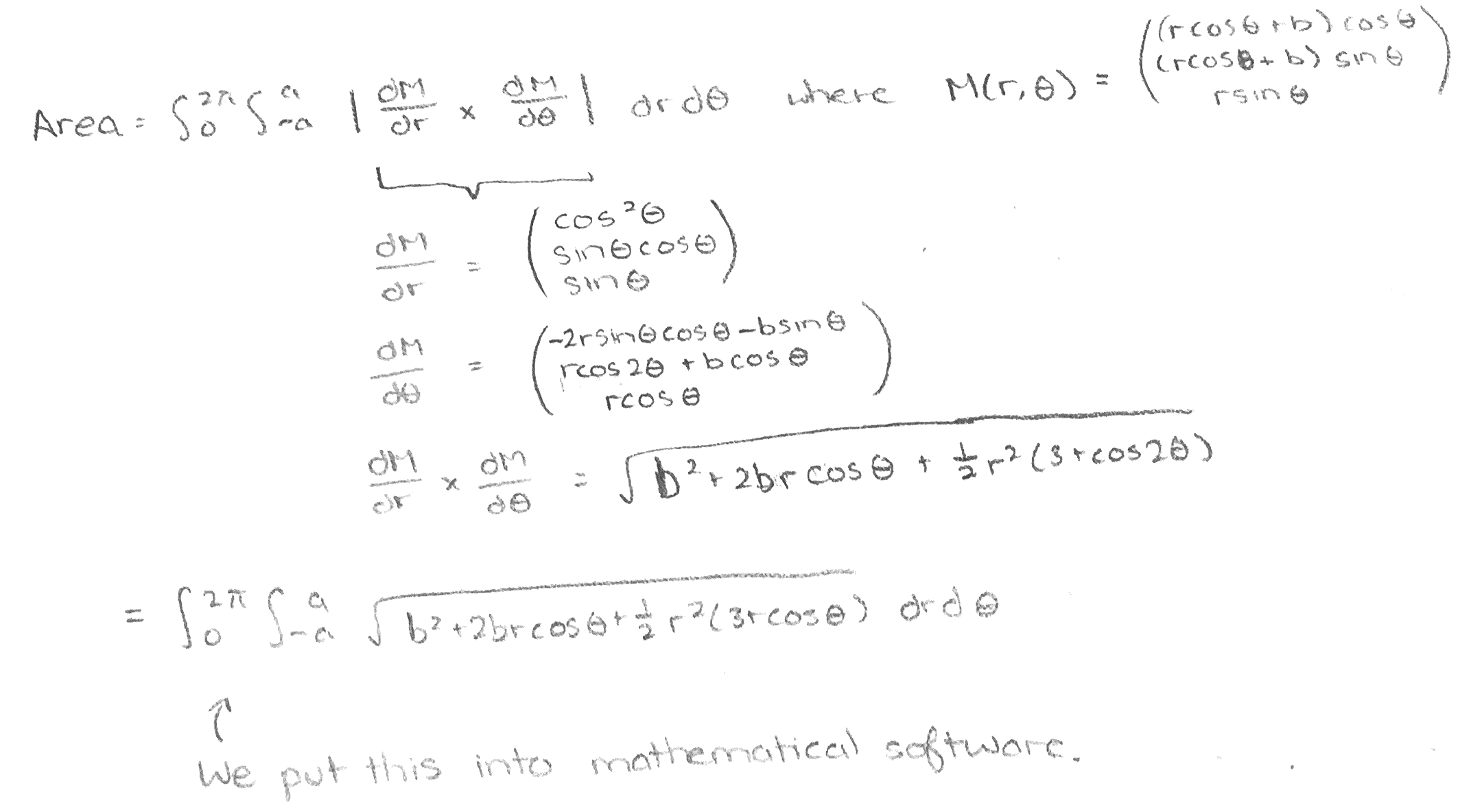(For a more thorough explanation of the calculus behind parametrizing a mobius strip and calculating surface area, see https://www.mathstat.dal.ca/~antoniov/bagelrings.html).

The surface area of a straight cut through the center of bagel is more straightforward. If you’ve ever split open a bagel the normal way, you’ll likely have noticed that the exposed bagel is simply a circle with a hole in the middle. Therefore, we can find this surface area just by taking the area of the outer circle (π(a+b)^2) and subtracting the area of the smaller circle (π(a-b)^2).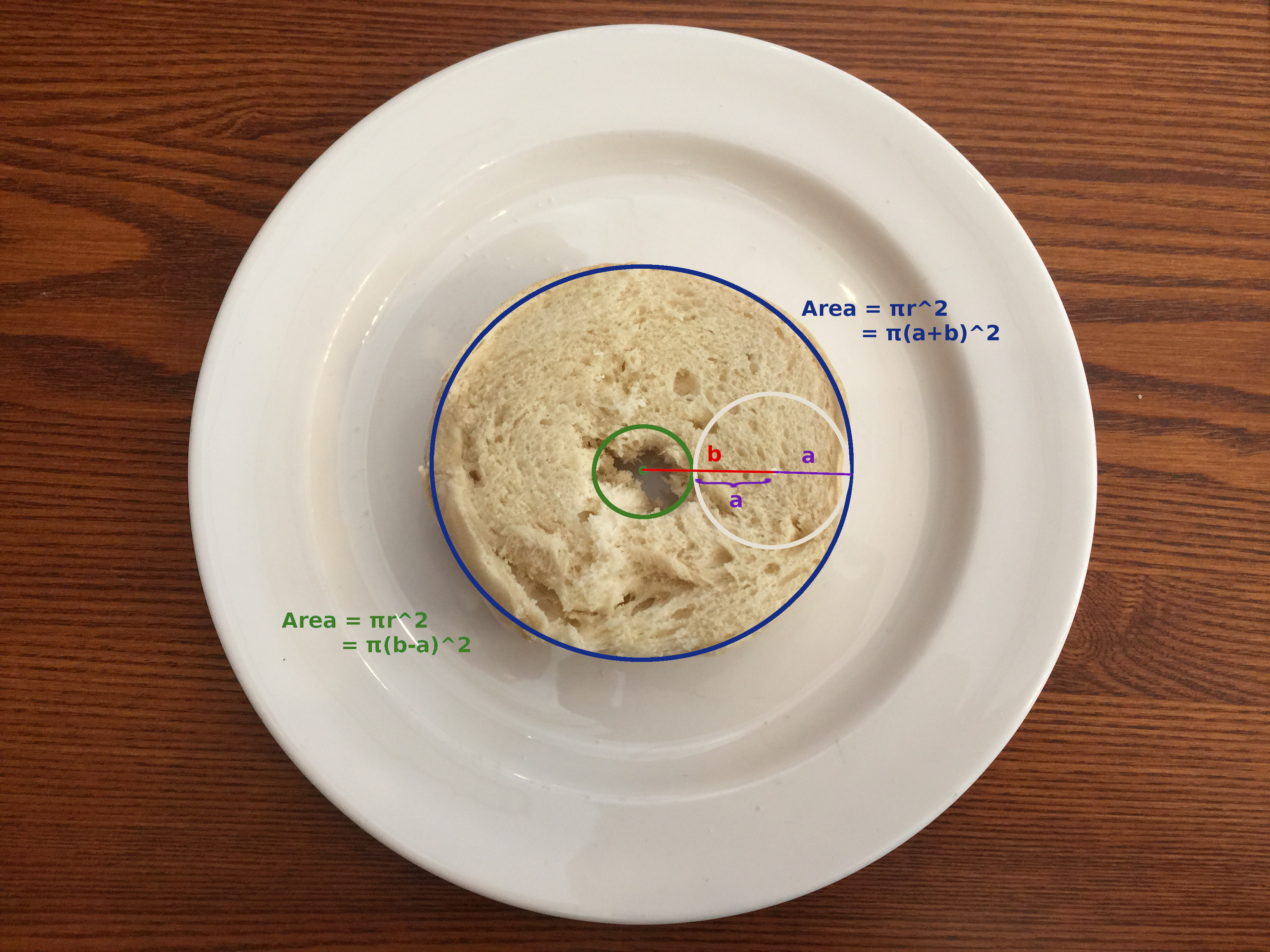Comparing the two areas using mathematical software, we find that the ratio of the surface area of the mobius cut to the surface area of the flat cut only changes when we change the ratio between a and b. Additionally, that ratio of surface areas is always greater than 1, so we can guarantee that the mobius strip will always give more surface area! However, the question of how much additional “bagelscape” we have for our cream cheese is dependent on the characteristics of the bagel shape. The greater a is in comparison to b, the greater the ratio of surface areas. That is, the fatter the bagel and the smaller the hole, the more benefit we get out of cutting our bagel using the mobius strip!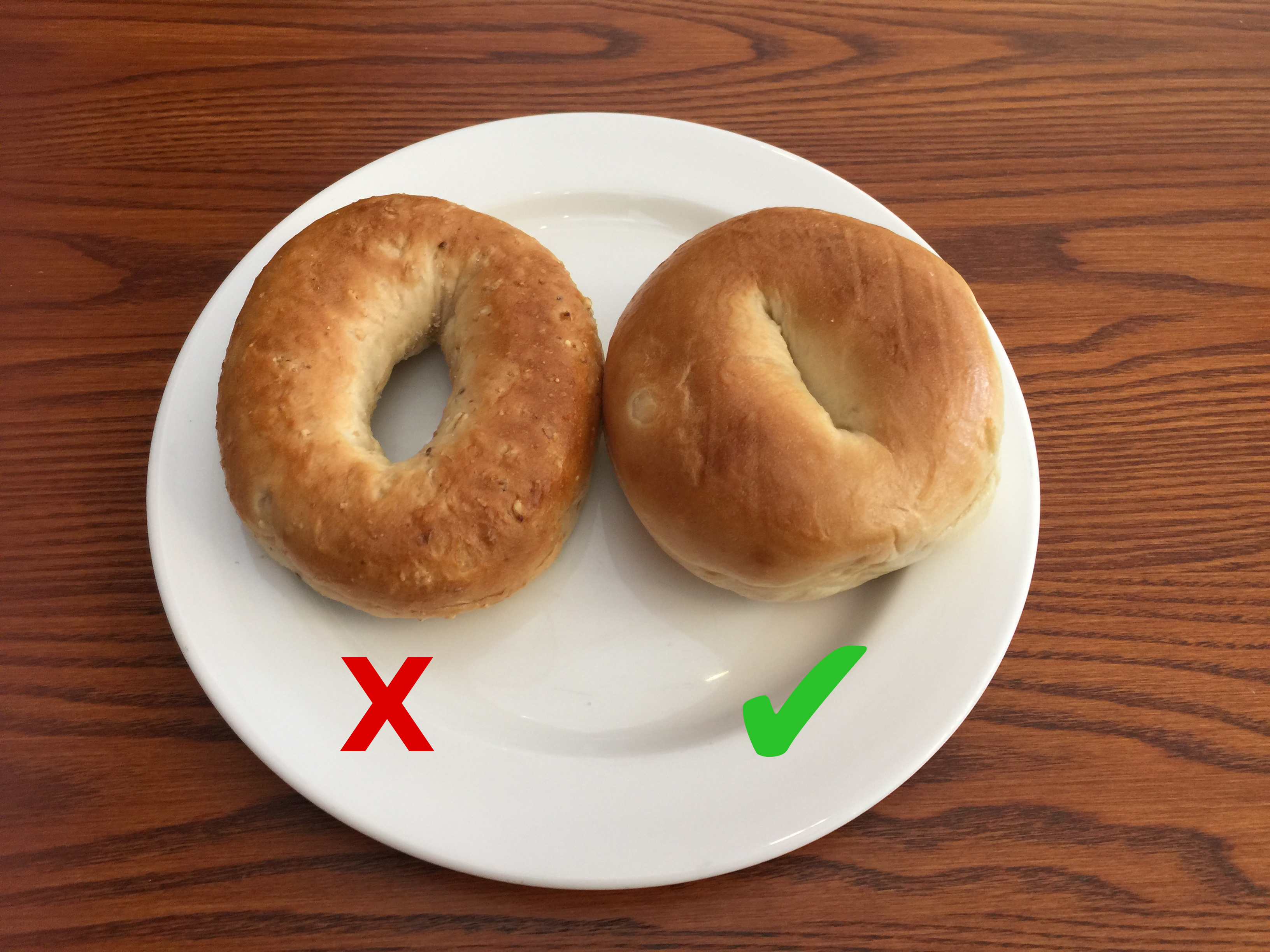In theory, the shape of the bagel on the left will give less significant gains in surface area from using a mobius cut while the shape of the one on the right will give more gains out of using a mobius cut.

However, we run into a significant physical reality check when we realize that for extremely thick bagels, the cut will not work because we cannot fit the bagel through its own center hole. Therefore, we restrict

2a < 2(b-a)

2a < 2b – 2a

4a < 2b

a < b/2

Therefore, referring to our previous diagram of the torus, the Mobius cut will only work for a<b/2, in which case the surface area gain is sadly only about 5% from our mathematical software analysis.

Resources and Acknowledgements:

Hart, George W. “Mathematically Correct Breakfast: How to slice a bagel into two linked halves.” https://www.georgehart.com/bagel/bagel.html

Vargas, Antonio R. “On calculating a ‘Mathematically Correct Breakfast’.” (2014). Dalhousie University. https://www.mathstat.dal.ca/~antoniov/bagelrings.html

Weisstein, Eric W. “Torus.” From MathWorld–A Wolfram Web Resource. http://mathworld.wolfram.com/Torus.html

## One thought on “The Math of Your Breakfast Bagel: Toruses and Mobius Strips”

1.thank you very good article